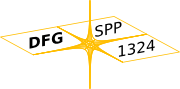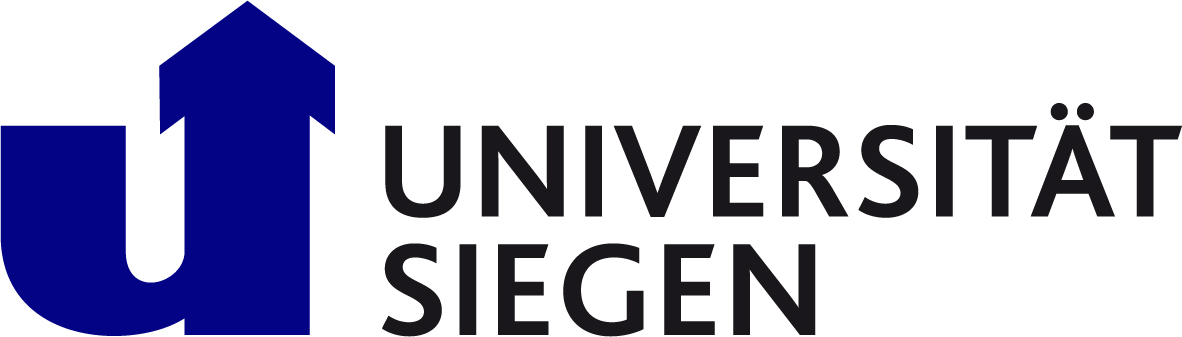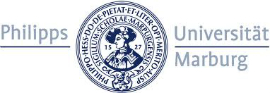Deutsche Forschungsgemeinschaft: DFG Priority Program 1324
Mathematical models of complex systems form the foundation for further technological developments in science, engineering and computational finance. A deeper understanding of the mathematical foundations as well as the development of new and efficient numerical algorithms are the main goals of this DFG Priority Program.

### ParticipantsUniversity Siegen: Prof. Dr. Thorsten Raasch
Major research topics of the numerics group in Siegen are: Adaptive wavelet and FEM schemes for operator equations, construction of wavelets, wavelet quadrature formulas, inverse problems, applications in engineering.Philipps University Marburg: Prof. Dr. Stephan Dahlke
The main research topics of the numerical analysis group in Marburg are: Adaptive wavelet and quarklet schemes for deterministic and stochastic operator equations, regularity theory for partial differential equations, construction of wavelets for general scalings, construction of (Banach) frames on manifolds, shearlet theory, coorbit theory, and mathematical modelling of microbiological systems.

Git tutorial: 15 minute tutorial to learn the basics of the versioning control system (VCS) git.

Git book: Pro Git book online resource. Extensive discourse about git.

Github: GitHub is a web-based Git or version control repository and Internet hosting service. The software of the WML is located there.

Github tutorial: 10 minute tutorial about github.

The NA Digest homepage: NA Digest is a collection of articles on topics related to numerical analysis and those who practice it.

The Netlib Repository: The Netlib Repository is a collection of mathematical software, papers, and databases.

Numerical Recipes: In partnership with Cambridge University Press, nr.com develops the Numerical Recipes series of books on scientific computing and related software products. They are one of the oldest continuously operating sites on the Web!